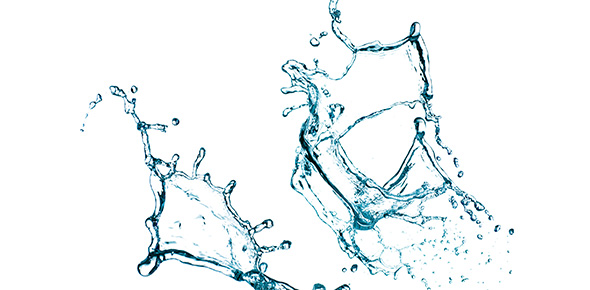# Freshwater Quiz (5 Questions)

10 Questions | Total Attempts: 525Settings• 1.
What is freshwater?
• A.

Water that makes up 3% of Earth's water composition

• B.

Water that is cold

• C.

Both A and B

• D.

None of the above

• 2.
What percentage of SALTWATER is there in Earth's water composition?
• A.

70%

• B.

3%

• C.

97%

• D.

100%

• E.

None of the above

• 3.
What percentage of FRESHWATER is melted and available to us?
• A.

60%

• B.

75%

• C.

3%

• D.

None of the above

• 4.
Where is most of our freshwater?
• A.

Trapped between mountains

• B.

Trapped in glaciers and ice caps

• C.

In an ancient and sacred pond in an unknown place

• D.

None of the above

• 5.
BONUS: Where is our saltwater found?
• A.

In oceans

• B.

In ponds

• C.

In glaciers and ice caps

• D.

In oceans and (some) lakes

• E.

None of the above (If you said this answer for the past 5 questions, you might want to study.)

• 6.
What is freshwater?
• A.

Water that makes up 3% of Earth's water composition

• B.

Water that is cold

• C.

Both A and B

• D.

None of the above

• 7.
What percentage of SALTWATER is there in Earth's water composition?
• A.

70%

• B.

3%

• C.

97%

• D.

100%

• E.

None of the above

• 8.
What percentage of FRESHWATER is melted and available to us?
• A.

60%

• B.

75%

• C.

3%

• D.

None of the above

• 9.
Where is most of our freshwater?
• A.

Trapped between mountains

• B.

Trapped in glaciers and ice caps

• C.

In an ancient and sacred pond in an unknown place

• D.

None of the above

• 10.
BONUS: Where is our saltwater found?
• A.

In oceans

• B.

In ponds

• C.

In glaciers and ice caps

• D.

In oceans and (some) lakes

• E.

None of the above (If you said this answer for the past 5 questions, you might want to study.)

Related TopicsBack to top